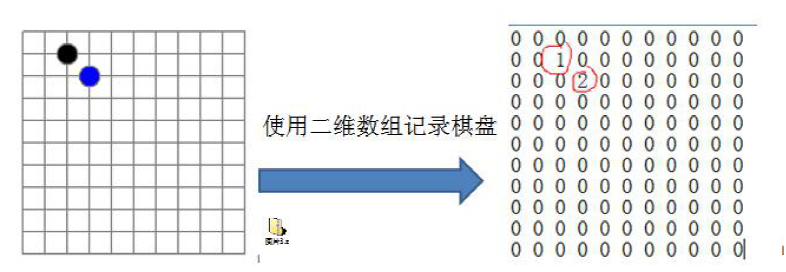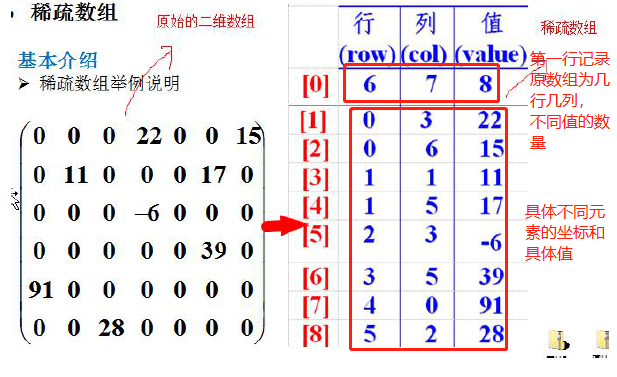# 一、实际需求

## 1.1 五子棋棋盘数据的存储

• 编写的五子棋程序中，有存盘和读盘的功能• 简易处理下，可以使用二维数组进行存储。

## 1.2 问题分析

• 由上图可见，若有效落子数据较少，二维数组的很多值是默认值0，因此记录了很多没有意义的数据。
• 优化这种情况，可使用稀疏数组

# 二、稀疏数组

## 2.1 基本介绍

• 当一个数组中的大部分元素为0，或者为同一个值的数组时，可以使用稀疏数组来保存该数组。
• 稀疏数组的处理方法：

1. 记录数组<font color="red">一共有几行几列有多少个不同</font>的值。
2. 把具有不同值的元素的行列记录在一个小规模的数组中，从而达到<font color="red">缩小程序规模</font>的目的。## 2.2 思路分析

### 2.2.1 二维数组to稀疏数组

1. 遍历原始数组，得到有效数据的个数`sum`
2. 根据sum创建系数数组`sparseArr`，规模为`int[sum+1]`
3. 将二维数组的有效数据存入到稀疏数组中

### 2.2.3 稀疏数组to二维数组

1. 先读取稀疏数组的第一行，根据第一行的数据，创建原始的二维数组
2. 再读取稀疏数组的后几行数据，赋值给二维数组即可

## 2.3 代码实现

``````public class SparseArray {

public static void main(String[] args) {
// 创建一个原始的二维数组 11 * 11
// 0: 表示没有棋子， 1 表示 黑子 2 表蓝子
int chessArr1[][] = new int;
chessArr1 = 1;
chessArr1 = 2;
chessArr1 = 2;
// 输出原始的二维数组
System.out.println("原始的二维数组~~");
for (int[] row : chessArr1) {
for (int data : row) {
System.out.printf("%d\t", data);
}
System.out.println();
}

// 将二维数组 转 稀疏数组的思
// 1. 先遍历二维数组 得到非0数据的个数
int sum = 0;
for (int i = 0; i < 11; i++) {
for (int j = 0; j < 11; j++) {
if (chessArr1[i][j] != 0) {
sum++;
}
}
}

// 2. 创建对应的稀疏数组
int sparseArr[][] = new int[sum + 1];
// 给稀疏数组赋值
sparseArr = 11;
sparseArr = 11;
sparseArr = sum;

// 遍历二维数组，将非0的值存放到 sparseArr中
int count = 0; //count 用于记录是第几个非0数据
for (int i = 0; i < 11; i++) {
for (int j = 0; j < 11; j++) {
if (chessArr1[i][j] != 0) {
count++;
sparseArr[count] = i;
sparseArr[count] = j;
sparseArr[count] = chessArr1[i][j];
}
}
}

// 输出稀疏数组的形式
System.out.println();
System.out.println("得到稀疏数组为~~~~");
for (int i = 0; i < sparseArr.length; i++) {
System.out.printf("%d\t%d\t%d\t\n", sparseArr[i], sparseArr[i], sparseArr[i]);
}
System.out.println();

//将稀疏数组 --》 恢复成 原始的二维数组
/*
*  1. 先读取稀疏数组的第一行，根据第一行的数据，创建原始的二维数组，比如上面的  chessArr2 = int 
2. 在读取稀疏数组后几行的数据，并赋给 原始的二维数组 即可.
*/

//1. 先读取稀疏数组的第一行，根据第一行的数据，创建原始的二维数组

int chessArr2[][] = new int[sparseArr][sparseArr];

//2. 在读取稀疏数组后几行的数据(从第二行开始)，并赋给 原始的二维数组 即可

for(int i = 1; i < sparseArr.length; i++) {
chessArr2[sparseArr[i]][sparseArr[i]] = sparseArr[i];
}

// 输出恢复后的二维数组
System.out.println();
System.out.println("恢复后的二维数组");

for (int[] row : chessArr2) {
for (int data : row) {
System.out.printf("%d\t", data);
}
System.out.println();
}
}

}``````
Last modification：November 25th, 2019 at 03:01 pm